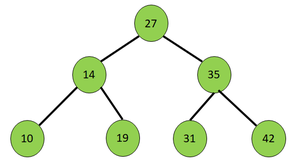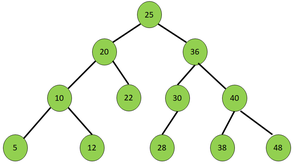Print nodes of a Binary Search Tree in Top Level Order and Reversed Bottom Level Order alternately

• Difficulty Level : Expert
• Last Updated : 22 Sep, 2020

Given a Binary Search Tree, the task is to print the nodes of the BST in the following order:

• If the BST contains levels numbered from 1 to N then, the printing order is level 1, level N, level 2, level N – 1, and so on.
• The top-level order (1, 2, …) nodes are printed from left to right, while the bottom level order (N, N-1, …) nodes are printed from right to left.

Examples:

Input:Output: 27 42 31 19 10 14 35
Explanation:
Level 1 from left to right: 27
Level 3 from right to left: 42 31 19 10
Level 2 from left to right: 14 35

Input:Output: 25 48 38 28 12 5 20 36 40 30 22 10

Recommended: Please try your approach on {IDE} first, before moving on to the solution.

Approach: To solve the problem, the idea is to store the nodes of BST in ascending and descending order of levels and node values and print all the nodes of the same level alternatively between ascending and descending order. Follow the steps below to solve the problem:

• Initialize a Min Heap and a Max Heap to store the nodes in ascending and descending order of level and node values respectively.
• Perform a level order traversal on the given BST to store the nodes in the respective priority queues.
• Print all the nodes of each level one by one from the Min Heap followed by the Max Heap alternately.
• If any level in the Min Heap or Max Heap is found to be already printed, skip to the next level.

Below is the implementation of the above approach:

C++

 // C++ program for the above approach    #include using namespace std;    // Structure of a BST node struct node {     int data;     struct node* left;     struct node* right; };    // Utility function to create a new BST node struct node* newnode(int d) {     struct node* temp         = (struct node*)malloc(sizeof(struct node));     temp->left = NULL;     temp->right = NULL;     temp->data = d;     return temp; }    // Function to print the nodes of a // BST in Top Level Order and Reversed // Bottom Level Order alternatively void printBST(node* root) {     // Stores the nodes in descending order     // of the level and node values     priority_queue > great;        // Stores the nodes in ascending order     // of the level and node values        priority_queue,                    vector >,                    greater > >         small;        // Initialize a stack for     // level order traversal     stack > st;        // Push the root of BST     // into the stack     st.push({ root, 1 });        // Perform Level Order Traversal     while (!st.empty()) {            // Extract and pop the node         // from the current level         node* curr = st.top().first;            // Stores level of current node         int level = st.top().second;         st.pop();            // Store in the priority queues         great.push({ level, curr->data });         small.push({ level, curr->data });            // Traverse left subtree         if (curr->left)             st.push({ curr->left, level + 1 });            // Traverse right subtree         if (curr->right)             st.push({ curr->right, level + 1 });     }        // Stores the levels that are printed     unordered_set levelsprinted;        // Print the nodes in the required manner     while (!small.empty() && !great.empty()) {            // Store the top level of traversal         int toplevel = small.top().first;            // If the level is already printed         if (levelsprinted.find(toplevel)             != levelsprinted.end())             break;            // Otherwise         else             levelsprinted.insert(toplevel);            // Print nodes of same level         while (!small.empty()                && small.top().first == toplevel) {             cout << small.top().second << " ";             small.pop();         }            // Store the bottom level of traversal         int bottomlevel = great.top().first;            // If the level is already printed         if (levelsprinted.find(bottomlevel)             != levelsprinted.end()) {             break;         }         else {             levelsprinted.insert(bottomlevel);         }            // Print the nodes of same level         while (!great.empty()                && great.top().first == bottomlevel) {             cout << great.top().second << " ";             great.pop();         }     } }    // Driver Code int main() {     /*     Given BST                                    25                               /     \                             20      36                            /  \      / \                           10   22   30 40                          /  \      /   / \                         5   12    28  38 48     */        // Creating the BST     node* root = newnode(25);     root->left = newnode(20);     root->right = newnode(36);     root->left->left = newnode(10);     root->left->right = newnode(22);     root->left->left->left = newnode(5);     root->left->left->right = newnode(12);     root->right->left = newnode(30);     root->right->right = newnode(40);     root->right->left->left = newnode(28);     root->right->right->left = newnode(38);     root->right->right->right = newnode(48);        // Function Call     printBST(root);        return 0; }

Output:

25 48 38 28 12 5 20 36 40 30 22 10

Time Complexity: O(V log(V)), where V denotes the number of vertices in the given Binary Tree
Auxiliary Space: O(V)

My Personal Notes arrow_drop_up
Recommended Articles
Page :